﻿ The Use of Cylindrical Lens - Hyperion Optics

# The Use of Cylindrical Lens

Cylindrical Lens can be used in a single axial convergence or divergence of the beam and found in optical measurement, laser scanning, spectroscopy, laser diode output beam shaping, the X-ray light microscopic imaging, and many other industries and fields have a wide range of applications.

Turn the quasi-direct light source into the line light source

L = 2(r0/f)(z+f)

It is the most extensive application of cylindrical lens. As shown in the figure below, The quasi-direct light source with radius r0 is irradiated into a concave cylindrical lens with a focal length of -f(The image is in order to illustrate the principle more clearly, so amplify the beam radius). The beam will diverge in half theta (theta = r0 / f). At this point, it can also be approximated as the divergence of the point source at the focal point -f. The distance to the back of the lens is z. The width of the line beam is 2r0 (ignoring the divergence of the  Gaussian-distributed beam spot), but the length of the line beam is changed

L = 2 (r0 / f) (z + f)

When z is greater than f, the expansion ratio approaches z/f, and the length of the line is proportional to z.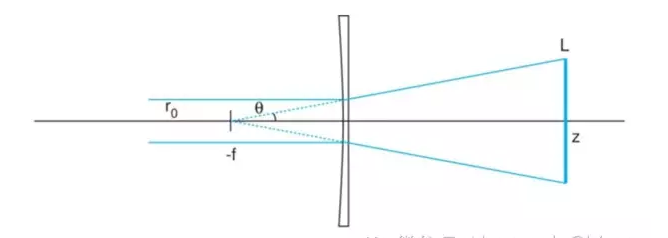If need in the z produces width is very narrow line light source, can be in the plane concave cylindrical lens front end or back end of a flat convex cylindrical lens focal length for z, with the orthogonal plane concave cylindrical lens place, to compress the beam width.

### 【 Quick Start】The focus and alignment of light

The diode outputs beam of collimation

The laser diode output beam diverges in an asymmetric form, and its quasi-direct work is more challenging. for example, to divergence angle theta. Theta 1 x 2 = 10 ° x 40 ° diode light source, if only use the standard spherical lens, and only in a single direction on collimating, another direction divergence or convergence will happen. Using a cylindrical lens that the problem is decomposed into two one-dimensional directions, through the combination of two orthogonal cylinder lens, two directions can be collimated at the same time.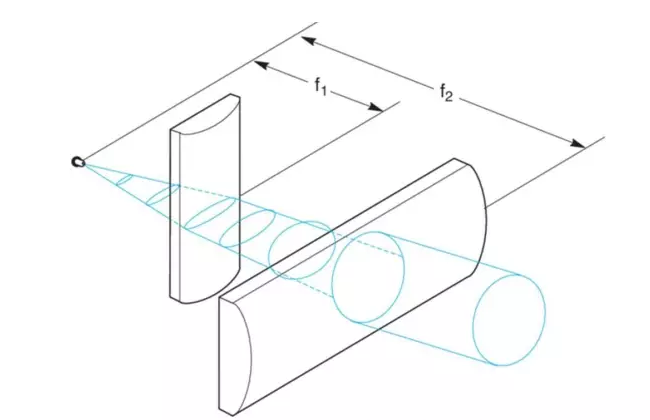The selection of the cylindrical lens and the installation of the light road should follow the below rules:

θ1/θ2 = 10°/40° = f1/f2

1)To make the spot symmetrical after the adjustment, the focal length ratio of the two cylindrical lenses is equivalent to the divergence angle.

Theta 1 / theta 2 = 10°/ 40° = f1 / f2

2）The laser diode can be approximated as a point source, to get the collimating output, The spacing between the two cylinders and the light source is equal to the focal length of the two.

3）The spacing between the main planes of the two cylinders should be equal to the difference between the focal length of the f2-f1, and the actual spacing between the two lenses is equal to BFL2 - BFL1. Like the spherical lens, the convex side of a cylindrical mirror should be directed toward a quasi-direct beam to minimize as much as possible.

d1 = 2f1(tan(θ2/2))

d2 = 2f2(tan(θ1/2))

4）Because the laser diode output beam diverges faster, we need to be careful to confirm that the spot size on each cylinder is no longer than the effective light aperture of the lens. Because the distance of the cylinder is equal to its focal length, the maximum spot width of each cylinder should be followed

D1 = 2f1 (theta 2/2)

D2 is equal to 2f2, the tangent of theta one half.

For example, Newport CKX012 (f1 = 12.7 mm, BFL1 = 7.49 mm) and CKX050 (f2 = 50.2 mm, BFL2 = 46.03 mm) the combination of cylinder lens, the spacing between the two lens on the plane for BFL2 - BFL1 = 38.54 mm. The diameter of the spot in the first cylindrical lens is

D1 = 2 (12.7 mm) tan (20 °) = 9.2 mm

The diameter of the light spot in the second cylinder is

D2 = 2 (50.2 mm) tan (5 °) = 8.8 mm

Although there is still a little asymmetry, the simple combinations of these two cylindrical lenses have greatly improved the quality of the beams.

Related Content
•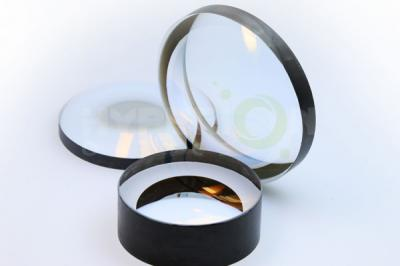## How Much Do You Know about the Telecentric Lens Application?

December 23, 20201. Application advantages of telecentric lensOne of the important advantages of telecentric lenses is that changes in object distance do not affect the magnification of the image. The telecentric lens...view
• ## What is the Collimation Function of Beam Expander

June 10, 20211. The collimation function of the beam expanderThe beam expander also has a collimating function, but the beam expander is aimed at the beam (beam with a certain divergence angle). Many lasers on the...view
•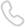Call us on:
+86-25-83307137
•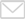Email us:
rfq@hypoptics.com
•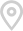R&D Center: Aoti Street #68 Building 4A 405 International R&D park, Nanjing
Manufacturing: Ruitai Rd#19 JiangNing District, Nanjing
• Sitemap
• R&D Center: Aoti Street #68 Building 4A 405 International R&D park, Nanjing
Manufacturing: Ruitai Rd#19 JiangNing District, Nanjing
• +86-25-83307137
• +86-25-86626312
• rfq@hypoptics.com
•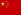• Hyperion Optics USA Inc.
1981 RT 27 STE 204
EDISON, NJ 08817
• +1 (908) 899-1918
• rfq-us@hypoptics.com
•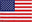•